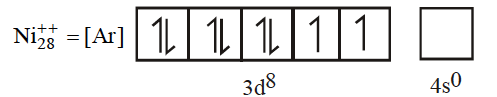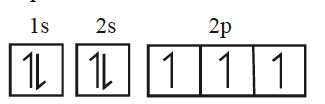NEET and AIPMT NEET Chemistry Structure of Atom MCQ Questions Solved

Which of the following sets of quantum numbers represent an impossible arrangement -

n   l    m     s

(A)  3   2   -2   (+)1/2

(B)  4   0   0    (-)1/2

(C)  3   2   -3   (+)1/2

(D)  5   3   0   (-)1/2

(C) If l=2, m $\ne$ -3

Difficulty Level:

• 11%
• 24%
• 58%
• 8%

How many unpaired electrons are present in Ni2+ cation (atomic number = 28)

(A) 0

(B) 2

(C) 4

(D) 6

(B) Two unpaired electrons are present in Ni++ (z=28) cationDifficulty Level:

• 17%
• 54%
• 24%
• 7%

Correct set of four quantum numbers for the outermost electron of rubidium (Z=37) is:

(A) 5, 0, 0, 1/2

(B) 5, 1, 0, 1/2

(C) 5, 1, 1, 1/2

(D) 6, 0, 0, 1/2

(A) Its configuration is 5s1

Difficulty Level:

• 59%
• 19%
• 17%
• 7%

Number of unparired electrons in 1S2 2S2 2P3 is -

1. 2

2. 0

3. 3

4. 1

(c) . In this type of electronic configuration the number of unpaired electrons are 3.Difficulty Level:

• 5%
• 7%
• 83%
• 7%

The quantum numbers of four electrons are given below.

n            $\mathcal{l}$           m          s

(1) Electron 1             3            0             0           -1/2

(2) Electron 2             4            0             1           1/2

(3) Electron 3            3             2             0           1/2

(4) Electron 4            3             1             0           -1/2

The correct order of decreasing energy of these electrons is -

(A) Electron 3 > Electron 1 > Electron 4> Electron 2

(B) Electron 4> Electron 2 > Electron 3 > Electron 1

(C) Electron 3 > Electron 2 > Electron 4 > Electron 1

(D) Electron 2 > Electron 4 > Electron 3 > Electron 1

(C) The correct order of decreasing energy will be electron 3 which has higher energy than 2 and in the order given.

Difficulty Level:

• 8%
• 20%
• 58%
• 17%

Which type of radiation is not emitted by the electronic structure of atoms:

(A) Ultraviolet light

(B) X-rays

(C) Visible light

(D) y-rays

(D) y-rays emission occurs due to radioactive change, a nuclear phenomenon.

Difficulty Level:

• 16%
• 13%
• 23%
• 50%

Of the following transitions in hydrogen atom, the one which gives an absorption line of lowest frequency is

(A) n=1 to n=2

(B) n=3 to n=8

(C) n=2 to n=1

(D) n=8 to n=3

(B)  Absorption line in the spectra arise when energy is absorbed i.e., electron shifts from lower to higher orbit, out of A and B, B will have the lowest frequency as this falls in the Paschen series.

Difficulty Level:

• 34%
• 27%
• 24%
• 17%

If uncertainty in the position of an electron is zero the uncertainty in its momentum will be

(A) < h/4$\mathrm{\pi }$

(B) > h/4$\mathrm{\pi }$

(C) zero

(D) infinite

(D) $∆$x x $∆$

if  $∆$x = 0, then  $∆$p will be infinite

Difficulty Level:

• 9%
• 20%
• 31%
• 43%

Which has highest e/m ratio:

1. He2+

2. H+

3. He1+

4. H

(B) Mass of His minimum.

Difficulty Level:

• 33%
• 26%
• 11%
• 32%

The maximum number of atomic orbitals associated with a principal quantum number 5 is

(A)9

(B)12

(C) 16

(D) 25

(D) The number of orbitals in a principle shell is n2= 52=25

Difficulty Level:

• 15%
• 14%
• 17%
• 55%